Case Based Questions (MCQ)

Class 10
Chapter 3 Class 10 - Metals and Non-Metals

## When a silvery grey powder of a solid (A) is  mixed with a powder of solid (B) no reaction  occurs. But if the mixture is ignited and lighted  using magnesium ribbon a reaction occurs with the evolution of a large amount of heat forming product  (C) which settles down as liquid metal and the  solid product (D) formed floats on the liquid (C).  (C) in solid form reacts with moisture to form rust.  The amount of heat generated during the reaction  is so high that the reaction is used in welding of  electric conductors, joints in railway tracks. Based  on this information, answer any four questions  from (a) to (e).

### (iv) A – Al and C - Cu

The reaction of a mixture of A and B in the presence of magnesium ribbon to form a liquid metal and oxide of D is the thermite reaction.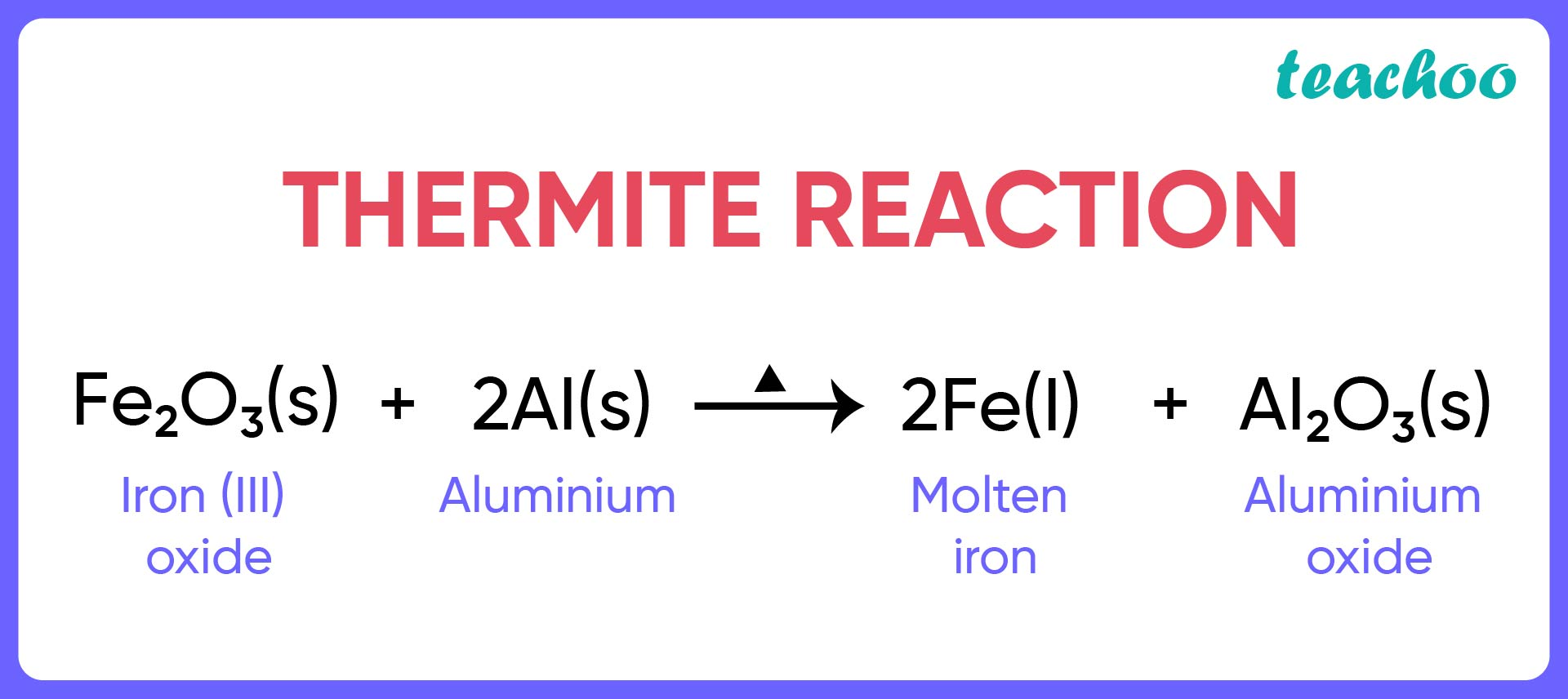According to this, A is Al because it is a grey colored metal and C is Fe because it forms rust in the presence of air and moisture.

So, the correct answer is (i) A - Al and C - Fe

### (iv) B – Al D – Fe

From the equation of thermite reaction,B is an oxide of Fe , and D of Aluminum .

### (iv) Metal oxides which show no reaction with  either acids or bases.

Ambhi means both. Therefore, the metal oxides which are able to react with both acid and base to form salt and water are known as amphoteric oxides.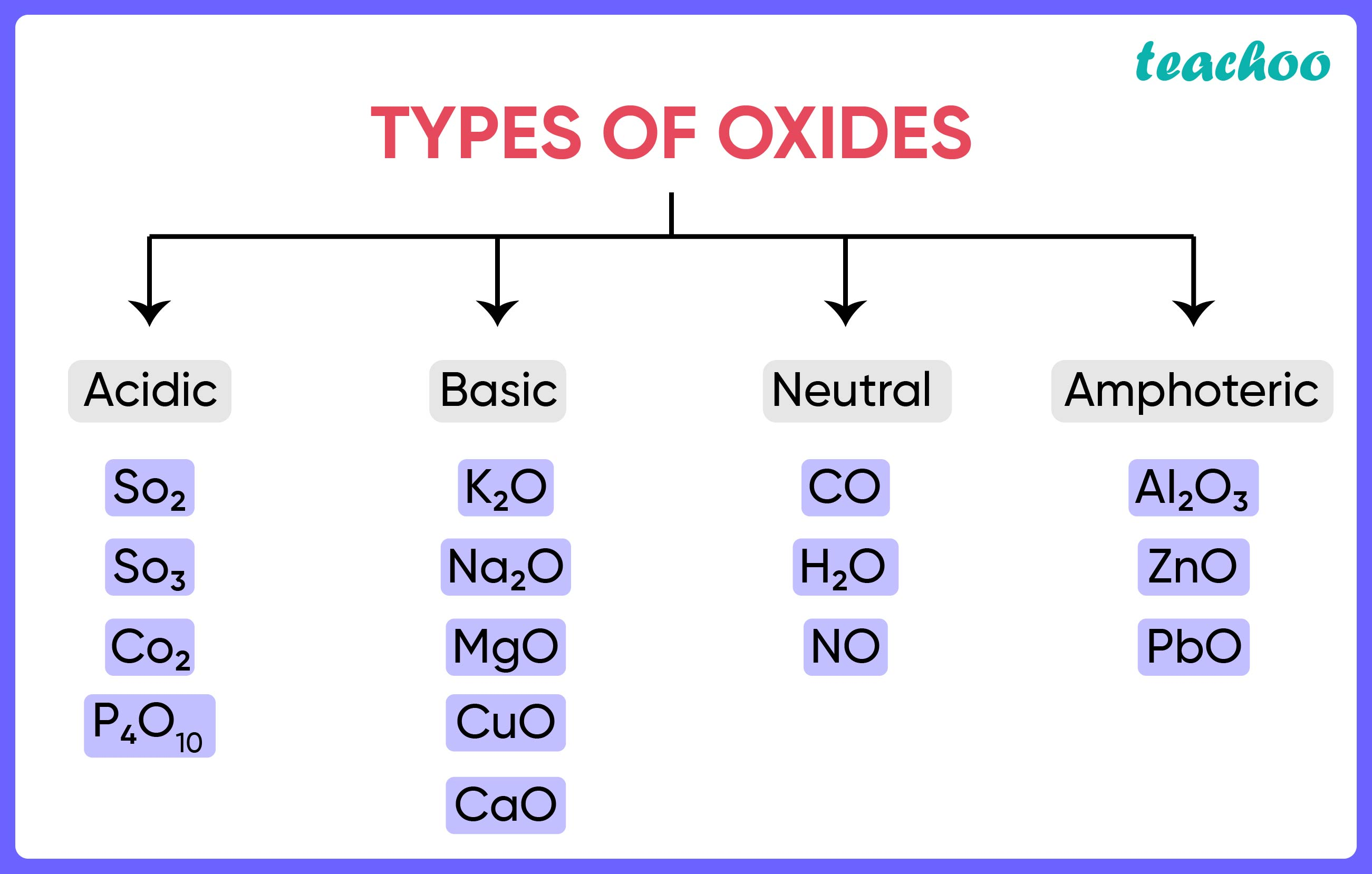So, the correct answer is (ii) Metal oxides which react with both acids as  well as bases.

### (iv) Neither of them.

Both aluminium oxide and zinc oxide are amphoteric in nature. Their reaction with acids and bases is given below:

Aluminium Oxide Acting as a Basic Oxide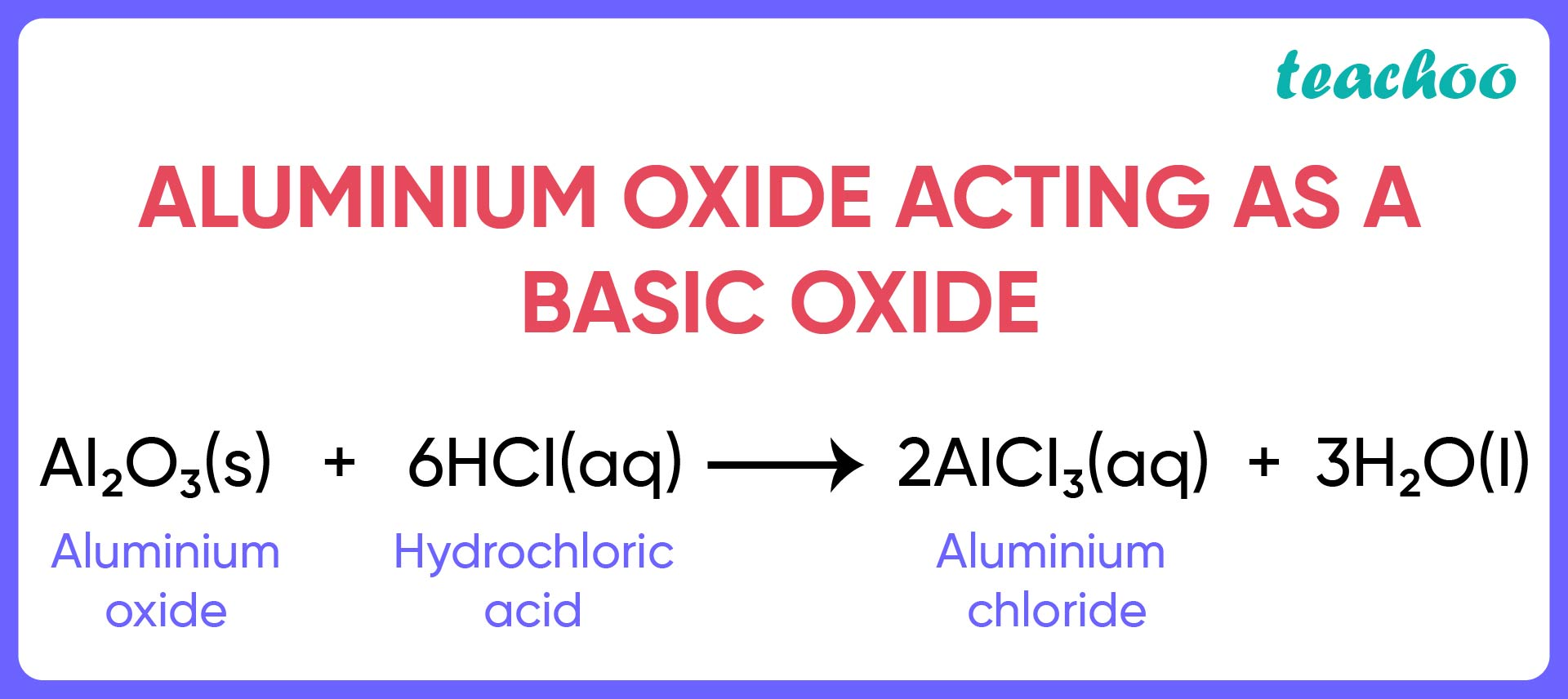Aluminium Oxide Acting as an Acidic Oxide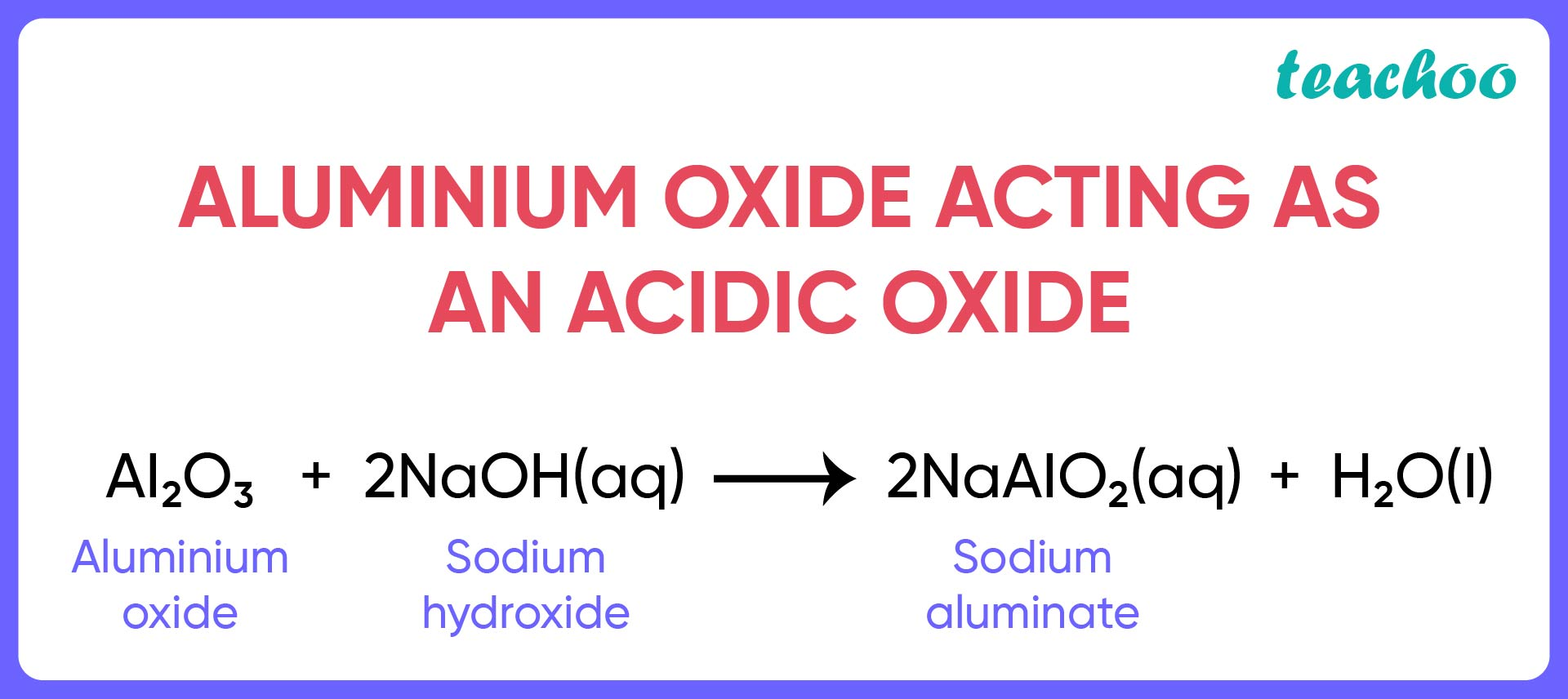Similarly, ZnO is also an amphoteric oxide.

Zinc Oxide Acting as a Basic Oxide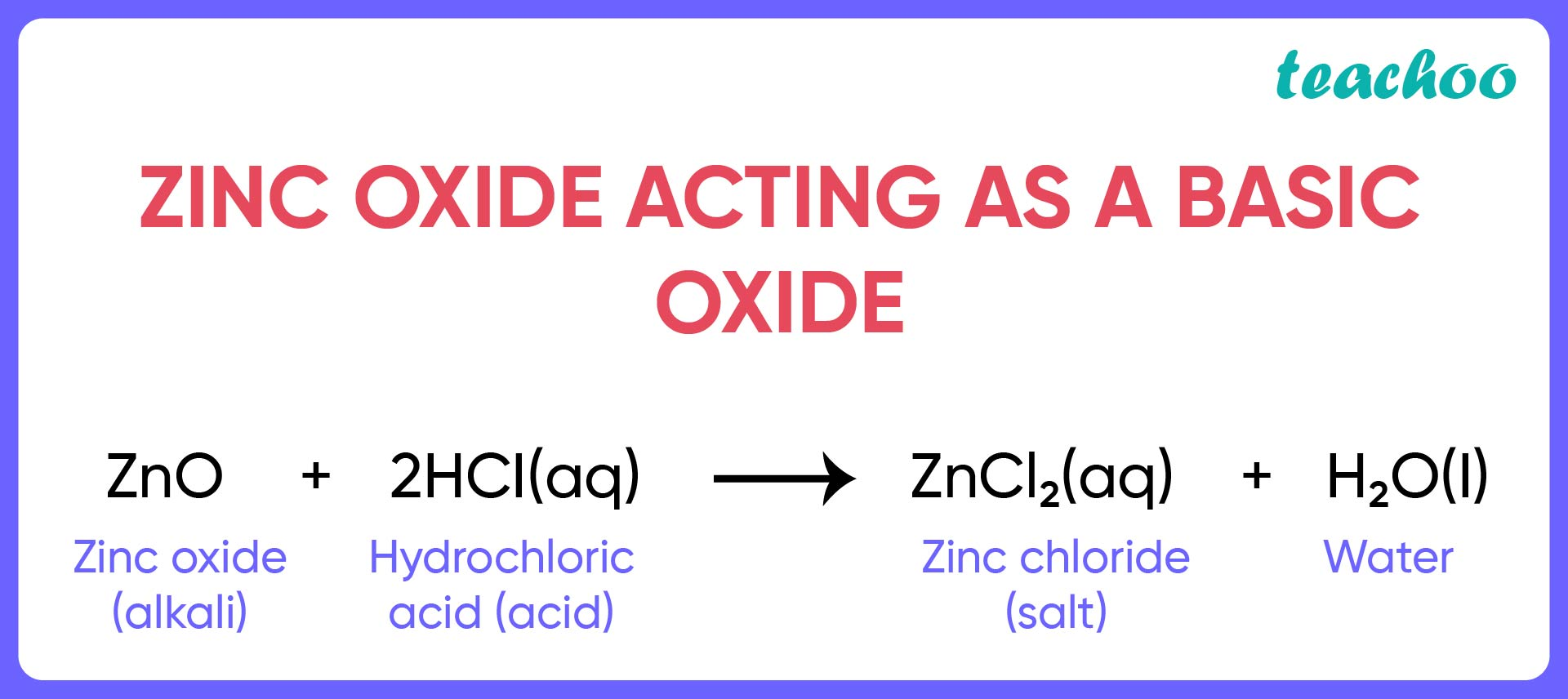Zinc Oxide Acting as an Acidic Oxide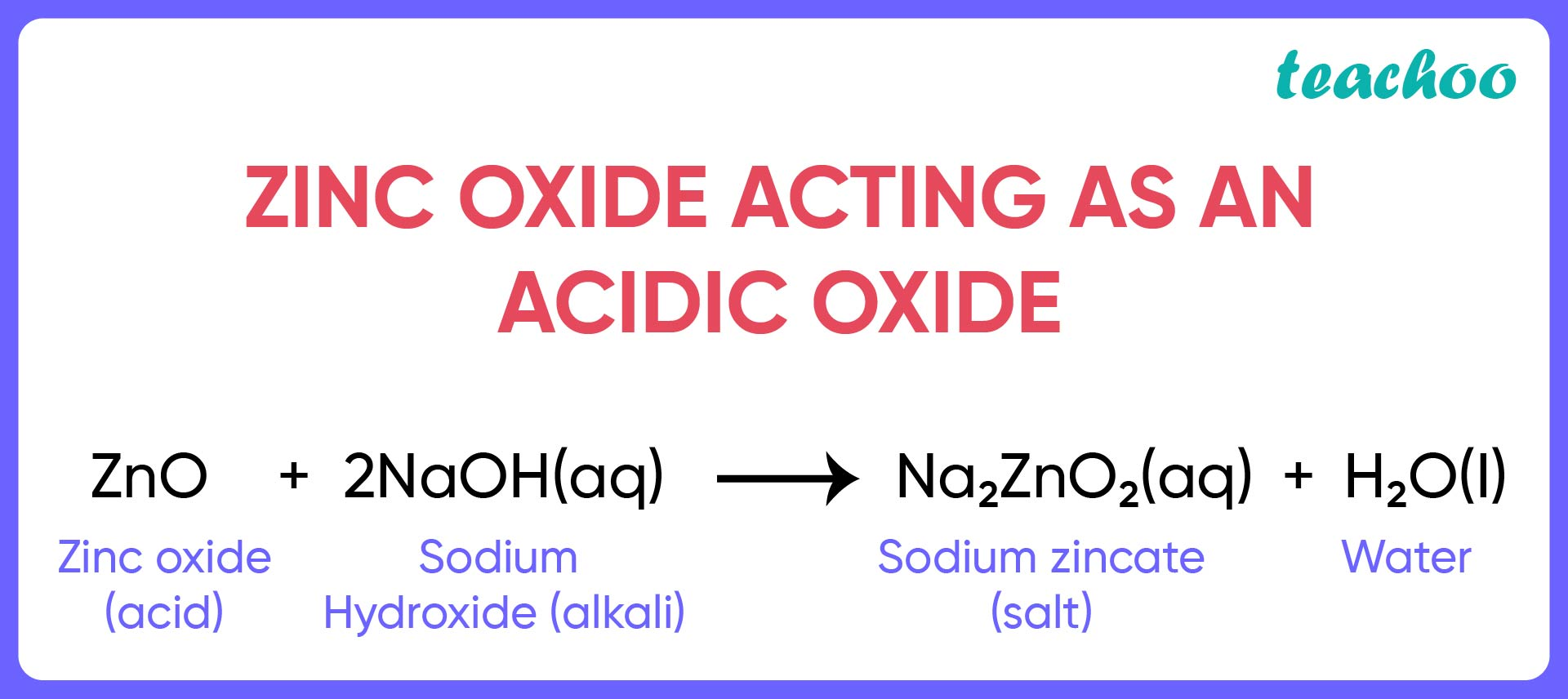So, the correct answer is (i)

### (iv) Precipitation reaction

Exo means out and thermic means heat . Hence, the reactions in which heat is generated are known as exothermic reactions .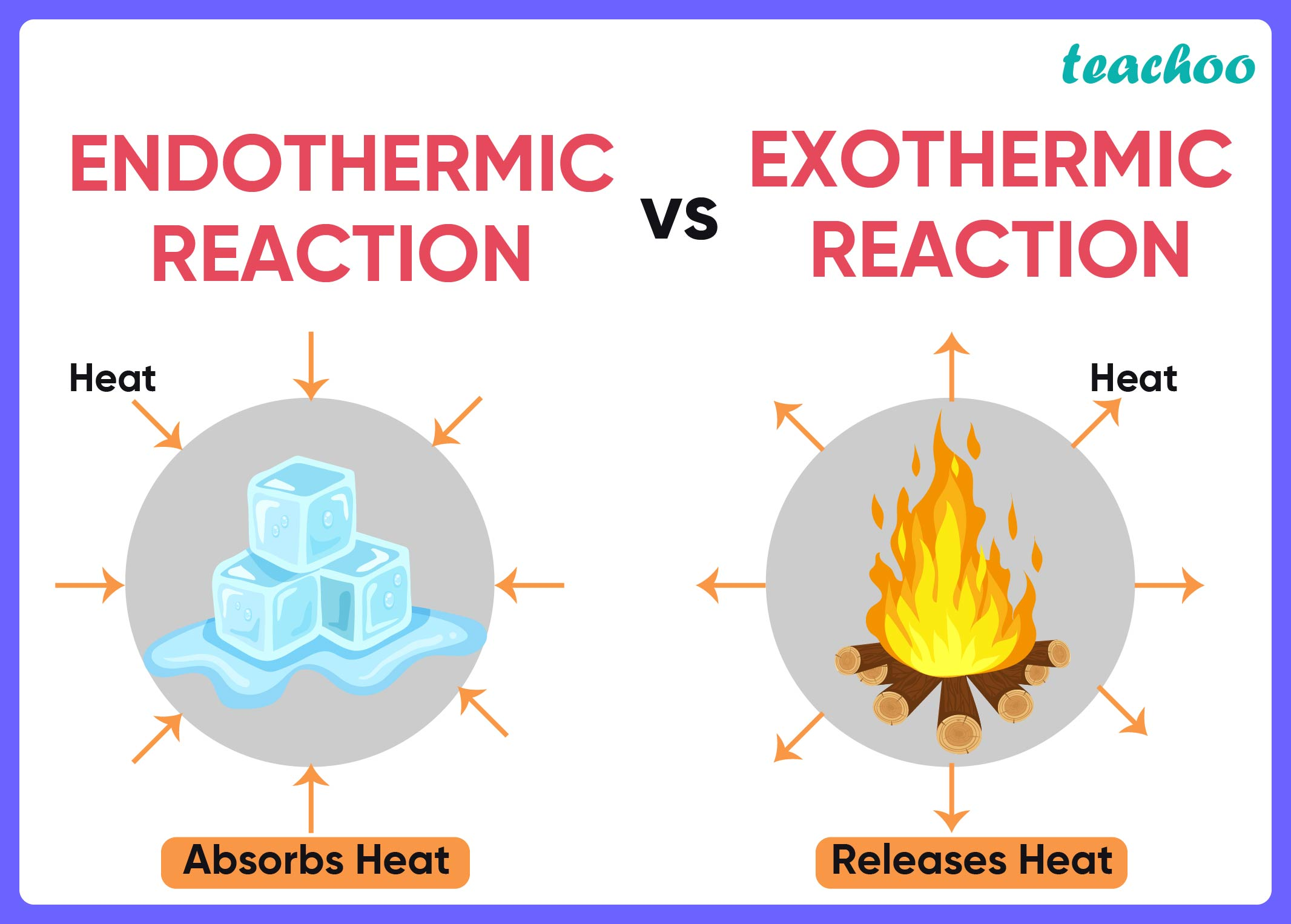So, the correct answer is (i) Exothermic reaction

Learn in your speed, with individual attention - Teachoo Maths 1-on-1 Class